Courses

# Solutions of Electricity (Page No- 41) - Physics By Lakhmir Singh, Class 10 Class 10 Notes | EduRev

## Class 10 : Solutions of Electricity (Page No- 41) - Physics By Lakhmir Singh, Class 10 Class 10 Notes | EduRev

The document Solutions of Electricity (Page No- 41) - Physics By Lakhmir Singh, Class 10 Class 10 Notes | EduRev is a part of the Class 10 Course Class 10 Physics Solutions By Lakhmir Singh & Manjit Kaur.
All you need of Class 10 at this link: Class 10

Lakhmir Singh Physics Class 10 Solutions Page No:41

Question 29:
A 5 V battery is connected to two 20 Ω resistors which are joined together in series.
(a) Draw a circuit diagram to represent this. Add an arrow to indicate the direction of conventional current flow in the circuit.
(b) What is the effective resistance of the two resistors ?
(c) Calculate the current that flows from the battery.
(d) What is the p.d. across each resistor ?

Solution :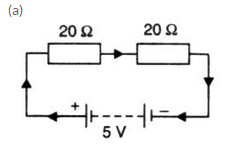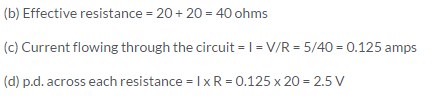Question 30:
The figure given below shows an electric circuit in which current flows from a 6 V battery through two resistors.
(a) Are the resistors connected in series with each other or in parallel ?
(b) For each resistor, state the p.d. across it.
(c) The current flowing from the battery is shared between the two resistors. Which resistor will have bigger share of the current ?
(d) Calculate the effective resistance of the two resistors.
(e) Calculate the current that flows from the battery.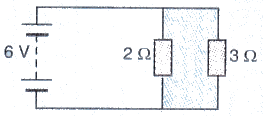Solution :

V = 6V. R= 2ohms. R2 = 3ohms

(a) Resistors are connected in parallel

(b) p.d. across each resistor is same and is equal to 6V.

(c) 2 ohms resistance have bigger share of current because of its lower resistance.

(d) Effective resistance = R

1/R = 1/2+1/3

1/R = S/6

R = 1.2ohms

(e) Current flowing through battery, l = V/R = 6/1.2 = 5amps

Question 31:
A 4 Ω coil and a 2 Ω coil are connected in parallel. What is their combined resistance ? A total current of 3 A passes through the coils. What current passes through the 2 Ω coil ?

Solution : 40 and 2Ω coil are connected in parallel. Combined resistance is R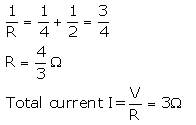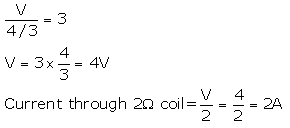Question 32:
(a) With the help of a circuit diagram, deduce the equivalent resistance of two resistances connected in series.
(b) Two resistances are connected in series as shown in the diagram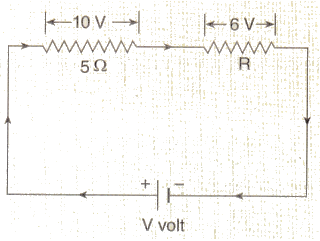(i) What is the current through the 5 ohm resistance ?
(ii) What is the current through R ?
(iii) What is the value of R ?
(iv) What is the value of V ?

Solution :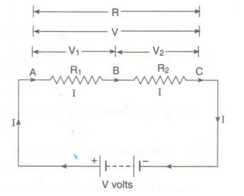(a) Fig shows two resistances R1 and R2 connected in series with a battery of V volts.

Let the p.d. across R1 is V1 and the p.d. across R2 is V2

S.t. V = V1 + V2 ....... (1)

Let the equivalent resistance be R and current flowing through whole circuit is I.

By Ohm's law,

V/I = R

V = I x R ---------(2)

Applying Ohm's law to both R1 end R2

V1 = I x R2 --------(3)

V2 = I X R2 ----- —(4)

From eqs. (1), (2), (3) and (4), we get

I x R = I x R1+ I x R2

I x R = I x (R1 + R2)

R = R1 + R2

(b)

(1) Current through 5Ω resisto r= 10/5 = 2A

Since 5Ω resistor and R are connected in series, so same current flows through them.

So, Current through R = 2A

(iii) V = IR

R = V/I = 6/2 = 3Ω

(iv) V = 10 + 6 = 16V

Question 33:
(a) With the help of a diagram, derive the formula for the resultant resistance of three resistors connected in series.
(b) For the circuit shown in the diagram given below :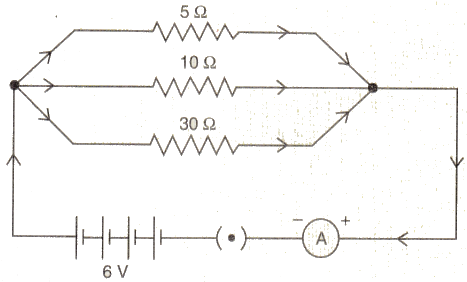Calculate :
(i) the value of current through each resistor.
(ii) the total current in the circuit.
(iii) the total effective resistance of the circuit.

Solution :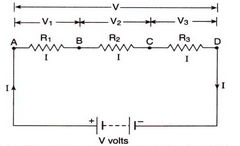(a) Ng shows three reSistances R1, R2 and R3 ecnnected in series with a battery of V volts.

Let the p.d. across R1, R2 and R3 is V1, V2 and V3 respectively.

s.t. V = V3 + V3 + V2  ----(1)

Let the equivalent resistance be R and arrent flowing through whole circuit is I.

By Ohm's law,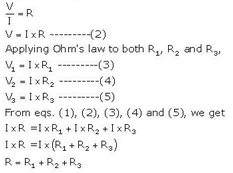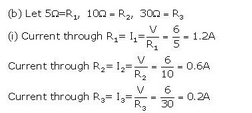(ii) Total current in the cirait = 1.2+0.6+0.2 = 24

(iii) Effective resistance R is given as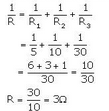Offer running on EduRev: Apply code STAYHOME200 to get INR 200 off on our premium plan EduRev Infinity!

94 docs

,

,

,

,

,

,

,

,

,

,

,

,

,

,

,

,

,

,

,

,

,

,

,

,

;